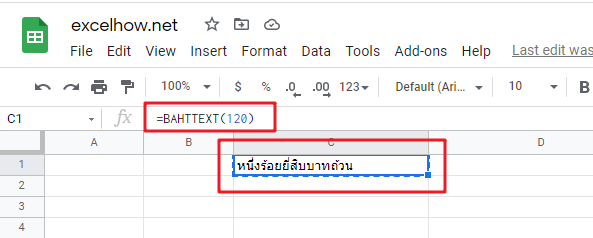# ExcelHow

This post will guide you how to use Google Sheets BAHTTEXT function with syntax and examples.

## Description

The Google Sheets BAHTTEXT function Converts a number to Thai text with the suffix Baht for integer values and Satang for decimal values.

The BAHTTEXT function is a build-in function in Google Sheets and it is categorized as a Text Function.

## Syntax

The syntax of the BAHTTEXT function is as below:

= BAHTTEXT(number)

Where the BAHTTEXT function arguments are:

• number -This is a required argument. It is the numeric value that you want to convert into Thai text.

Note:

• If the supplied number argument is not recognized as a numeric value in google sheets, the BAHTTEXT function returns #VALUE! Error message.

## Google Sheets BAHTTEXT Function Examples

The below examples will show you how to use google sheets BAHTTEXT function to convert a number into Thai text with the suffix “Baht”.

#1 To convert a number in Cell B1 to Thai text, just using the following formula:

`= BAHTTEXT(B1)  //it returns “หนึ่งร้อยยี่สิบบาทถ้วน”`